Question

# Suppose you want to prepare a buffer with a pH of 4.35 using formic acid. What...

Suppose you want to prepare a buffer with a pH of 4.35 using formic acid. What ratio of [sodium formate]/[formic acid] do you need to make this buffer? Formic acid has a Ka of 1.8x10-4.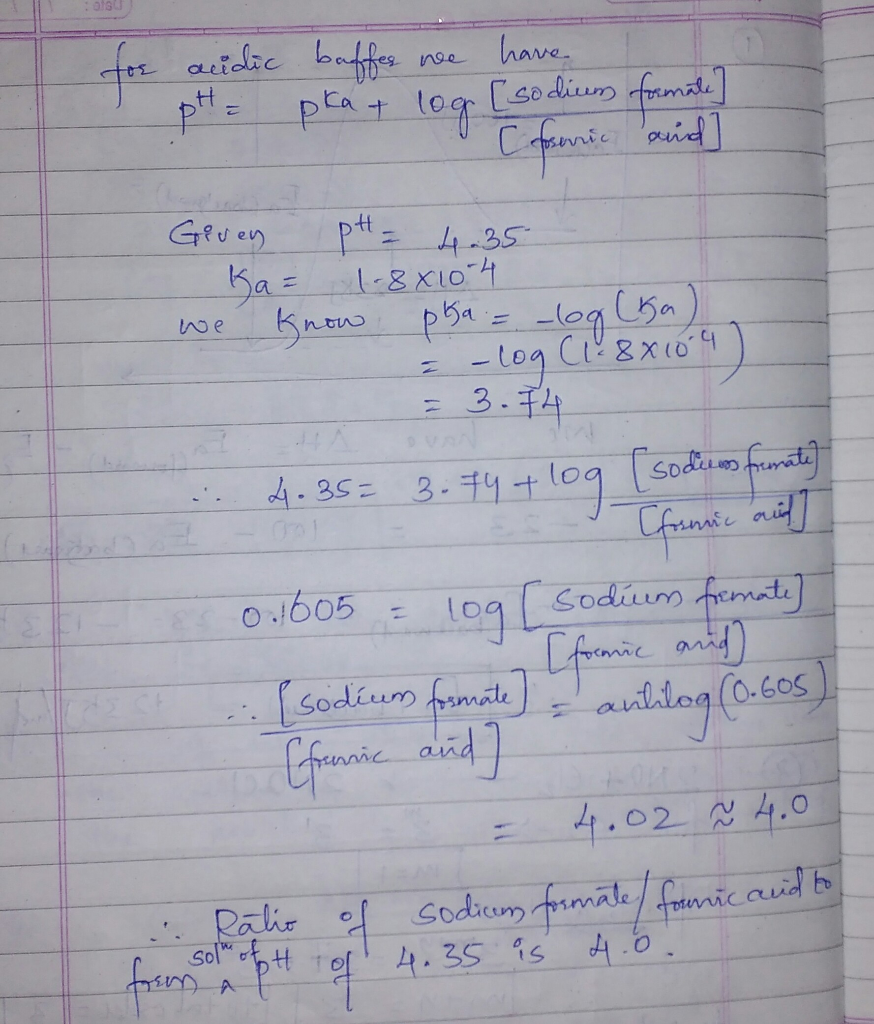Thank you vmuch...Thank you vmuch...

#### Earn Coins

Coins can be redeemed for fabulous gifts.

Similar Homework Help Questions
• ### You combine 0.75 moles formate and 0.85 moles formic acid to make a buffer solution. The...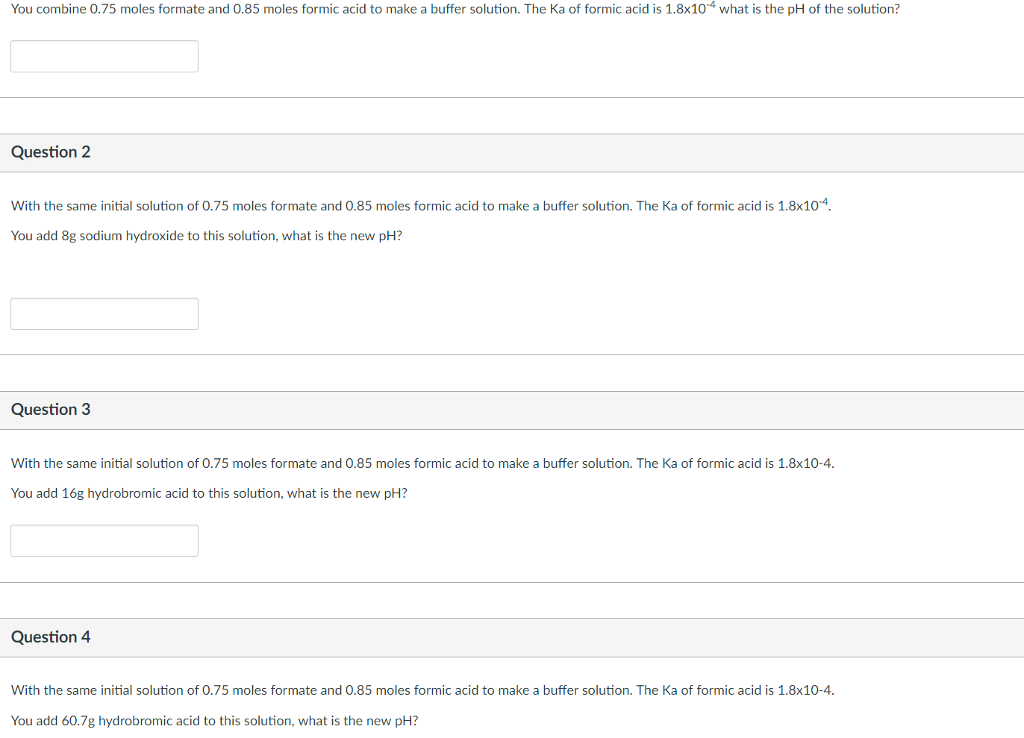You combine 0.75 moles formate and 0.85 moles formic acid to make a buffer solution. The Ka of formic acid is 1.8xl0'4 what is the pH of the solution? With the same initial solution of 0.75 moles formate and 0.85 moles formic acid to make a buffer solution. The Ka of formic acid is 1.8xl0'4. You add 8g sodium hydroxide to this solution, what is the new pH? With the same initial solution of 0.75 moles formate and 0.85 moles...

• ### You and your lab partner must prepare a 1.0 L buffer of formic acid at pH...

You and your lab partner must prepare a 1.0 L buffer of formic acid at pH 3.5. Your lab partner started the process and has already massed out 0.23 g of formic acid (MM 46 g/mol). How many moles of sodium formate do you need to complete this buffer. The pKa of formic acid is 3.75.

• ### 2. A Student wants to prepare 250.0 mL of pH 4.1 buffer solution. He is planning...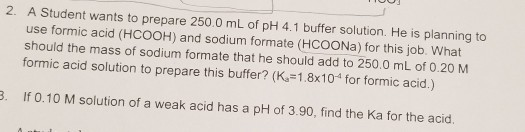2. A Student wants to prepare 250.0 mL of pH 4.1 buffer solution. He is planning to use formic acid (HCOOH) and sodium formate (HCOONa) for this job. What should the mass of sodium formate that he should add to 250,0 mL of 0.20 M formic acid solution to prepare this buffer? (K =1.8x10 for formic acid.) 3. If 0.10 M solution of a weak acid has a pH of 3.90, find the Ka for the acid.

• ### QUESTION 3 To make a buffer of formic acid (Ka = 1.8 x 10-4) with a...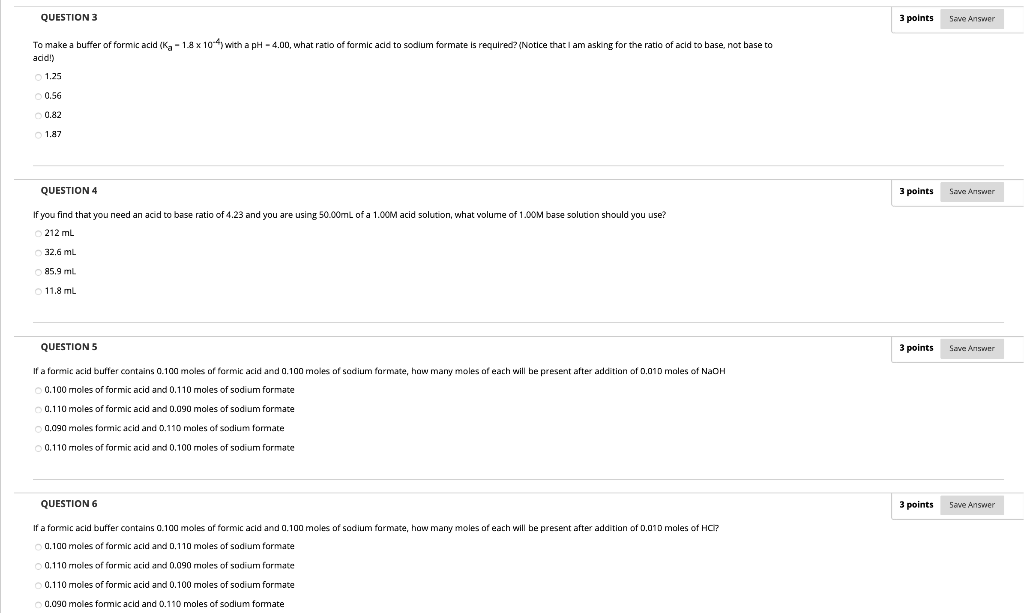QUESTION 3 To make a buffer of formic acid (Ka = 1.8 x 10-4) with a pH = 4.00, what ratio of formic acid to sodium formate is required? (Notice that I am asking for the ratio of acid to base, not base to acid!) a) 1.25 b) 0.56 c) 0.82 d) 1.87 QUESTION 4 If you find that you need an acid to base ratio of 4.23 and you are using 50.00mL of a 1.00M acid solution, what volume...

• ### PLEASE COMPLETE ALL ANSWER. SHOW ALL WORK. For questions 10 and 11. You need a buffer...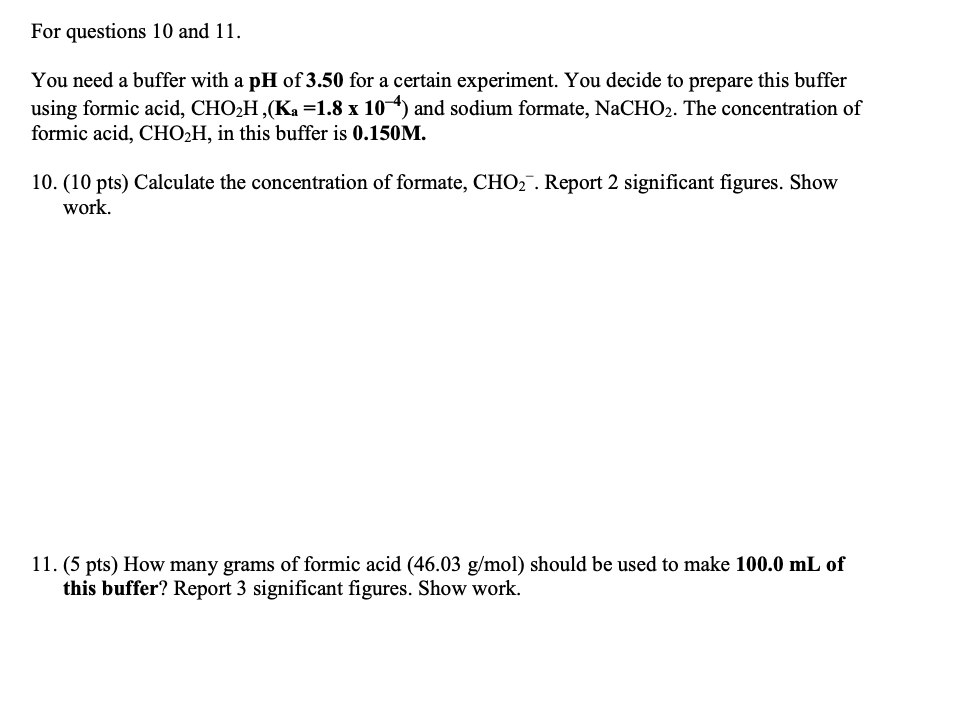PLEASE COMPLETE ALL ANSWER. SHOW ALL WORK. For questions 10 and 11. You need a buffer with a pH of 3.50 for a certain experiment. You decide to prepare this buffer using formic acid, CHO2H,(Ka =1.8 x 104) and sodium formate, NaCHO2. The concentration of formic acid, CHO2H, in this buffer is 0.150M. 10. (10 pts) Calculate the concentration of formate, CHO2. Report 2 significant figures. Show work. 11. (5 pts) How many grams of formic acid (46.03 g/mol) should...

• ### a buffer solution with ph of 4.63 is prepared with 0.14m formic acid and ___m sodium...

a buffer solution with ph of 4.63 is prepared with 0.14m formic acid and ___m sodium formate. The ka of formic acid is 1.8*10^-4.

• ### Starting from 0.5 M formic acid and solid sodium formate. Describe how to prepare 5 L of Formate buffer, pH = 4, Ka =1....

Starting from 0.5 M formic acid and solid sodium formate. Describe how to prepare 5 L of Formate buffer, pH = 4, Ka =1.78×10-4

• ### 1. formic acid, HFor, has a Ka value equal to about 1.8x10^-4. A student is asked...

1. formic acid, HFor, has a Ka value equal to about 1.8x10^-4. A student is asked to prepare a buffer having a pH of 3.55 from a solution of formic acid and a solution of sodium formate having the same molarity. How many milliliters of the NaFor solution should she add to 20mL of the HFor solution to make the buffer? 2. how many mL of 0.10M NaOH should the student add to 20mL 0.10M HFor if she wishedto prepare...

• ### A solution buffered at pH 3.90 is needed for a reaction. Would formic acid (HCOOH =...

A solution buffered at pH 3.90 is needed for a reaction. Would formic acid (HCOOH = HA) and its salt, sodium formate, NaHCO2 = NaA), make a good choice for this buffer? If so, what ratio of moles of A- and HA is needed? Use the Henderson Hasselbalch Equation. Ka formic acid = 1.8 x 10-4

• ### Calculate the pH of a buffer solution that is 0.30 M formic acid (HCO2H) and 0.50...

Calculate the pH of a buffer solution that is 0.30 M formic acid (HCO2H) and 0.50 M sodium formate (HCO2Na). Ka of HCO2H is 1.8 x 10-4